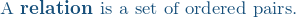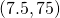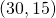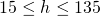# London EyeThe London Eye is a giant ferris wheel opened to the public in the year 2000 on the river Thames, London, England.

It takes about 30 minutes to complete a rotation. When boarding, the capsule is about 15 meters above the ground. At the top, the capsule is 135 meters above the ground.

Let’s define a relation as follows:is the height of the capsule above the ground at time.

In this case the independent variable is time, and the dependent variable is height. Both are numerical, so we can graph this relation.

The domain will be time, between 0 minutes and 30 minutes.

The range will be ‘height of capsule above the ground’, and will have values between 15 and 135 meters.

The relation will be: (time, height) as follows:

At zero minutes, the capsule will be 15 meters above the ground:Quarter way around: 7.5 minutes, 75 meters (half way between 15 m and 135 m):Half way around: 15 minutes, 135 meters (top):Three quarters way around: 22.5 minutes, 75 meters:All the way around 30 minutes: 15 meters above the ground:The relation (time after departure, height above the ground) includes the ordered pairsHowever, there are infinitely many points in this relation as time is continuous – we can find the height of a passenger cabin at time 10 minutes, or time 10.2 minutes, or 10.000002 minutes etc. Therefore the height of the capsule has been calculated at every 5 seconds.

# Observations:

The values on the horizontal axes represent the domain,.

The values on the vertical axis represent the range,.

The capsule gains and loses height more quickly at some times and more slowly at other times.

In the first 2.5 minutes (that would be the first 30° rotation), it gains very little height – about 10 m.

Between 5 minutes and 7.5 minutes it gains about 35 m.

In the same quantity of time, it the height gain is different depending on where the capsule is on the circle.

This is because it takes the same time to travel around equal sections of the circle – the bottom and top of the circle are relatively flat and so around these times not much height is gained or lost. The left and right sides are steep, so when in these positions, a lot of height is gained or lost quickly.

Back to Unit 5 Project